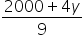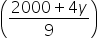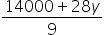Mathematics
Easy

Question

# The ratio of incomes of two persons is 9:7, and the ratio of their expenditure is 4:3. If each of them manages to save \$2000 per month, find their monthly expenditure?

## \$16000, \$12000\$8000, \$10000\$11000, \$20000     None of the aboveHint:

## The correct answer is: \$16000, \$12000

### Step 1 of 1:We have given the ratio of incomes of two persons is 9:7, and the ratio of their expenditure is 4:3. If each of them manages to save \$2000 per monthLet the income be x and expenditure be ySaving per month = \$2000Then9x – 4y = 2000………………….. 17x – 3y = 2000……………… 2From 19x – 4y = 20009x = 2000 + 4yx =Substituting x in 2nd equation,7- 3y = 2000- 3y = 200014000+ 28y – 27y = 1800014000 + 1y = 18000y = 40004y = 4 x 4000=160003y = 3 x 4000= 12000

The substitution method is the algebraic method to solve simultaneous linear equations

### Related Questions to study#### With Turito Foundation.#### Get an Expert Advice From Turito.